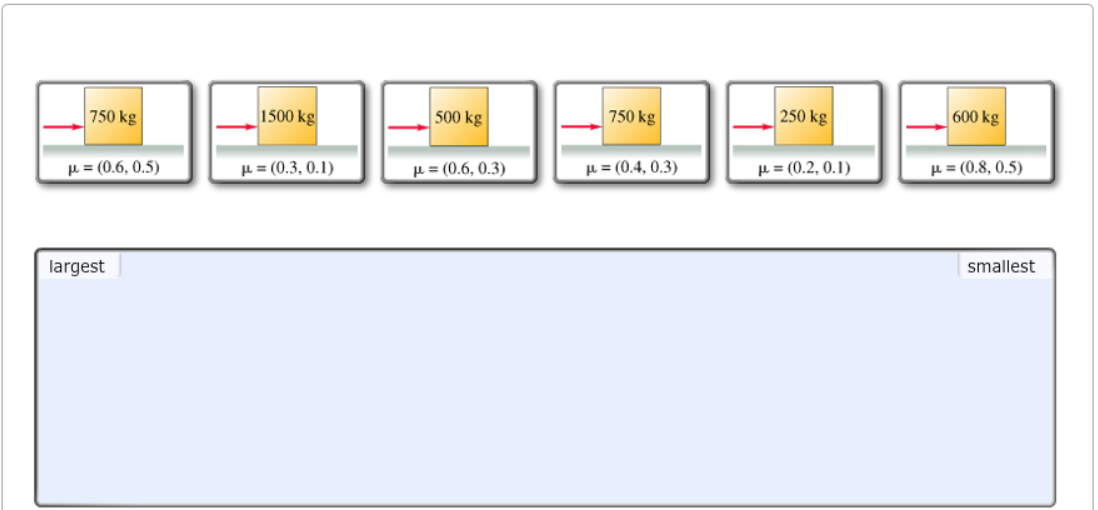# Problem: Rank the crates on the basis of the static frictional force acting on them. Rank from largest to smallest. To rank items as equivalent, overlap them. Assume the applied force is the maximum that can be applied without the crate moving.

###### FREE Expert Solution

Friction force:

$\overline{)\begin{array}{rcl}{\mathbf{f}}& {\mathbf{=}}& \mathbf{\mu }\mathbf{N}\\ {\mathbf{f}}& {\mathbf{=}}& \mathbf{\mu }\mathbf{m}\mathbf{g}\end{array}}$

Ranking based on static friction:

fs = μsmg

For 1, fs = (0.6)(750)(9.81) = 4415 N

89% (267 ratings)###### Problem Details

Rank the crates on the basis of the static frictional force acting on them. Rank from largest to smallest. To rank items as equivalent, overlap them. Assume the applied force is the maximum that can be applied without the crate moving.